INCREASING AND DECREASING FUNCTIONS Analysis

One of the first applications of the derivative can be found by studying how a function increases and decreases.

You should already be familiar with the graphs of increasing and decreasing functions.

 1.Derivative and increase 2.Calculating the intervals 3.Applications

1. THE RELATION BETWEEN THE DERIVATIVE AND WHETHER THE FUNCTION INCREASES OR DECREASES

Let f be a function with a derivative.

We can say that a function y=f(x) INCREASES at xo when there is a neighbourhood of xo such that:

• if x£xo then f(x)£f(xo) and if xo£x then f(xo)£f(x)

 Change the value of x in the window or drag the red point with the mouse to check that in this case the sign of [f(x)-f(xo)] = the sign of [x-xo]

If f is a function with a derivative then: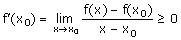If the function has a derivative and increases at xo then f'(xo) ³ 0

We can say that a function y=f(x) DECREASES at xo when there is a neighbourhood of xo such that:

• if x£xo then f(x)³f(xo) and if xo£x then f(xo)³f(x)

 Change the value of x in the window (click on the arrows or drag the red point with the mouse) to check that now the sign of [f(x)-f(xo)] ¹ the sign of [x-xo]

In this case: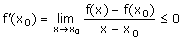If a function has a derivative and decreases at xo then f'(xo) £ 0

Look carefully at the graph of the function y=f(x) and its derivative y=f'(x) in the window.

 Change the value of x in the window and check that f increases when x<1 and decreases when x>1

Remember that the derivative of a function at a point is the same as the gradient of the tangent to the curve of the function at that point.

• What is the gradient of the tangent to the curve like when the function is increasing? What about when the function is decreasing?

• What is the relation between the sign of the derivative and whether the function is increasing or decreasing?

 When f'(xo)>0 then f is increasing at xo When f'(xo)<0 then f is decreasing at xo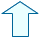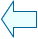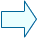María José García CebrianSpanish Ministry of Education. Year 2001